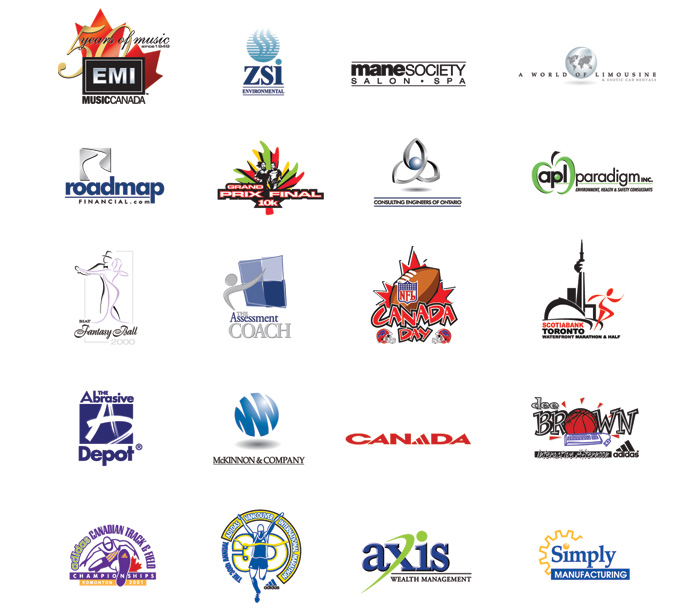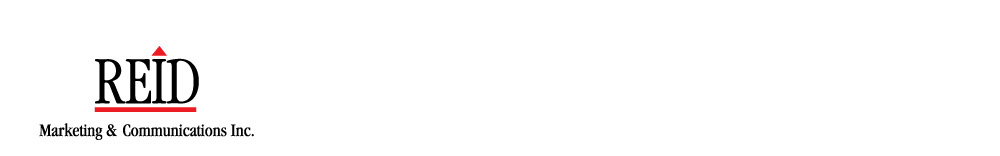# <!-- function MM_swapImgRestore() { //v3.0 var i,x,a=document.MM_sr; for(i=0;a&&i<a.length&&(x=a[i])&&x.oSrc;i++) x.src=x.oSrc; } function MM_preloadImages() { //v3.0 var d=document; if(d.images){ if(!d.MM_p) d.MM_p=new Array(); var i,j=d.MM_p.length,a=MM_preloadImages.arguments; for(i=0; i<a.length; i++) if (a[i].indexOf("#")!=0){ d.MM_p[j]=new Image; d.MM_p[j++].src=a[i];}} } function MM_findObj(n, d) { //v4.01 var p,i,x; if(!d) d=document; if((p=n.indexOf("?"))>0&&parent.frames.length) { d=parent.frames[n.substring(p+1)].document; n=n.substring(0,p);} if(!(x=d[n])&&d.all) x=d.all[n]; for (i=0;!x&&i<d.forms.length;i++) x=d.forms[i][n]; for(i=0;!x&&d.layers&&i<d.layers.length;i++) x=MM_findObj(n,d.layers[i].document); if(!x && d.getElementById) x=d.getElementById(n); return x; } function MM_swapImage() { //v3.0 var i,j=0,x,a=MM_swapImage.arguments; document.MM_sr=new Array; for(i=0;i<(a.length-2);i+=3) if ((x=MM_findObj(a[i]))!=null){document.MM_sr[j++]=x; if(!x.oSrc) x.oSrc=x.src; x.src=a[i+2];} } //--> <!-- a:link { text-decoration: none; } a:visited { text-decoration: none; } a:hover { text-decoration: none; } a:active { text-decoration: none; } body { margin-left: 0px; margin-top: 0px; margin-right: 0px; margin-bottom: 0px; } -->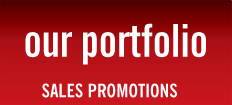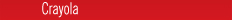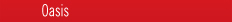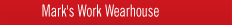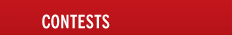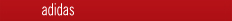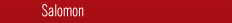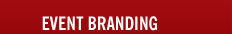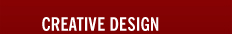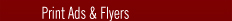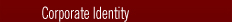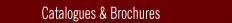CORPORATE IDENTITY

To develop effective logos, the design must be appropriate for the business it identifies. This is accomplished by working closely with the client and through in-depth research into the industry involved. These strategic objectives helps facilitate the development of dynamic, visually appealing logos that effectively represent both the brand and the company.